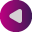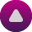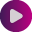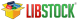`Floating Point Constants  mikroC PRO for PIC32 Language Reference > Lexical Elements > Tokens > Constants > ` ``

## Floating Point Constants

A floating-point constant consists of:

• Decimal integer
• Decimal point
• Decimal fraction
• `e` or `E` and a signed integer exponent (optional)
• Type suffix: `f` or `F` or `l` or `L` (optional)

Either decimal integer or decimal fraction (but not both) can be omitted. Either decimal point or letter `e` (or `E`) with a signed integer exponent (but not both) can be omitted. These rules allow conventional and scientific (exponent) notations.

Negative floating constants are taken as positive constants with an unary operator minus (-) prefixed.

The mikroC PRO for PIC32 limits floating-point constants to the range ±2.2250E * 10-308 .. ±6.80564774407 * 1038.

If there is a need for a floating-point constant that exceeds these limits, user should add `l` or `L` suffix, making the constant a `long double` type.

Here are some examples:

```0.       // = 0.0
-1.23    // = -1.23
23.45e6  // = 23.45 * 10^6
2e-5     // = 2.0 * 10^-5
3E+10    // = 3.0 * 10^10
.09E34   // = 0.09 * 10^34
2.E100L  // = 2.0 * 10^100```

The mikroC PRO for PIC32 floating-point constants are of the type `double`. Note that the mikroC PRO for PIC32’s implementation of ANSI Standard considers `float` and `double` to be the same type.

Find them on